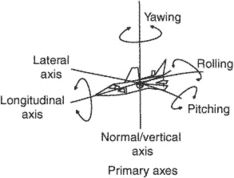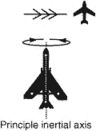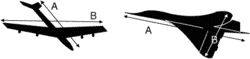# principal axis

(redirected from Principal axes)
Also found in: Dictionary, Thesaurus, Medical.

## principal axis

[′prin·sə·pəl ′ak·səs]
(crystallography)
The longest axis in a crystal.
(engineering acoustics)
A reference direction for angular coordinates used in describing the directional characteristics of a transducer; it is usually an axis of structural symmetry or the direction of maximum response.
(mathematics)
One of a set of perpendicular axes such that a quadratic function can be written as a sum of squares of coordinates referred to these axes.
For a conic, a straight line that passes through the midpoints of all the chords perpendicular to it.
For a quadric surface, the intersection of two principal planes.
(mechanics)
One of three perpendicular axes in a rigid body such that the products of inertia about any two of them vanish.
(optics)
McGraw-Hill Dictionary of Scientific & Technical Terms, 6E, Copyright © 2003 by The McGraw-Hill Companies, Inc.

## principal axisPrimary axesIn the illustration on the left, line A is the principal axis while in the illustration on the right, line B is the principal axis. The moment of inertia is maximum around these axes in these two cases.
i. The lateral, vertical, and longitudinal axes.
ii. The axis of relative wind.
iii. The principal inertial axis: that line passing through the center of gravity in a plane of symmetry about which a long, slender body tends to rotate when rolling.
iv. A rectilinear axis in the plane of a cross section of a structural member about which the moment of inertia is the maximum.
References in periodicals archive ?
(ii) With respect to the principal axis III, a unidirectional control torque is generated whenever control torques are applied around the principal axes I and II.
Correia, "The principal axes transformation-a method for image registration," Journal of Nuclear Medicine, vol.
For the two obtained VOIs, the principal axes are extracted as descriptors to be used in the feature correspondence step.
In Figure 4(a), it is observed that the first three principal components represent 78.6% variation of the data, reason why it is decided to represent the data using these three components as principal axes of representation; this is visualized in Figure 4(b), with projections of the original data on the three principal components.
The component form of the constitutive model (26) with respect to the Lagrangian principal axes is
The direction of the principal axes of the birefringence was essentially constant over the sample length, indicated by the fact that linear light polarization along this direction was well preserved.
The two analyses are equivalent in the sense that each has the same total inertia, the same dimensionality and the same decomposition of inertia into principal inertias along principal axes.
Three principal axes (a, b, c represent the longest, medium and shortest axis, respectively, as shown in Fig.
Polynomial strength criteria show formal approximation of experimental data in the coordinates of principal axes, however.
Having initially examined both German and Soviet plans for the summer campaign and the preliminaries to operations on the Stalingrad axis such as the Khar'kov battle of May 1942, the subsequent chapters look initially at operations on the principal axes toward Stalingrad.

Site: Follow: Share:
Open / Close